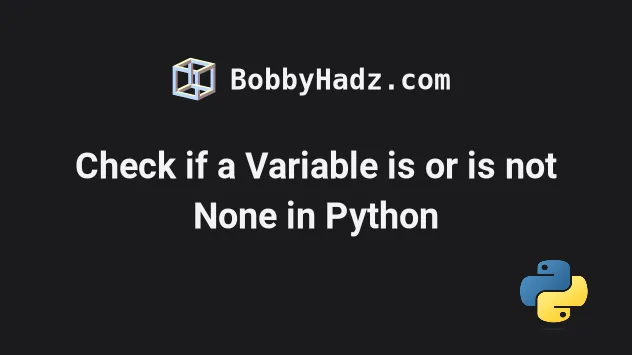# Check if a variable is None in PythonWed Jun 15 20223 min readPhoto by Katie McBroom

## Check if a variable is None in Python#

Use the `is` operator to check if a variable is None in Python, e.g. `if my_var is None:`. The `is` operator returns `True` if the values on the left-hand and right-hand sides point to the same object and should be used when checking for singletons like `None`.

main.py
```Copied!```my_var = None

# ✅ Check if variable is None
if my_var is None:
# 👇️ this runs
print('variable is None')

# ✅ Check if variable is NOT None
if my_var is not None:
print('variable is not None')
``````
The first `if` statement checks if the variable stores a `None` value, and the second - if the variable doesn't store a `None` value.

You should use the `is` operator when you need to check if a variable stores a `None` value.

When we use `is`, we check for the object's identity.

The pep 8 style guide mentions that comparison to singletons like `None` should always be done with `is` or `is not`, and never the equality operators.

Use the equality operators (equals `==` and not equals `!=` ) when you need to check if a value is equal to another value, e.g. `'hello' == 'hello'`.

Here is an example that better illustrates checking for identity (`is`) vs checking for equality (`==`).

main.py
```Copied!```my_first_list = ['a', 'b', 'c']

my_second_list = my_first_list # 👈️ same list as above

print(my_first_list is my_second_list)  # 👉️ True

print(my_first_list == my_second_list)  # 👉️ True
``````

We declared 2 variables that store the same list.

We set the second variable to the first, so both variables point to the same `list` object in memory.

Now, let's create a shallow copy of the list and assign it to the second variable.

main.py
```Copied!```my_first_list = ['a', 'b', 'c']

my_second_list = my_first_list.copy() # 👈️ copy created

print(my_first_list is my_second_list)  # 👉️ False

print(my_first_list == my_second_list)  # 👉️ True
``````
Notice that the identity check failed. Even though the two lists store the same values, in the same order, they point to different locations in memory (they are not the same object).

When we use the double equals operator, Python calls the `__eq__()` method on the object.

That is `x==y` calls `x.__eq__(y)`. In theory this method could be implemented in an unpredictable way, so checking for `None` with the `is` operator is more direct.

You can use the id() function to get the identity of an object.

main.py
```Copied!```my_first_list = ['a', 'b', 'c']
print(id(my_first_list))  # 👉️ 139944523741504

my_second_list = my_first_list.copy()
print(id(my_second_list))  # 👉️ 139944522293184

print(id(my_first_list) == id(my_second_list))  # 👉️ False
``````
The function returns an integer, which is guaranteed to be unique and constant for the object's lifetime.

The `id()` function returns the address of the object in memory in CPython.

If the two variables refer to the same object, the `id()` function will produce the same result.

main.py
```Copied!```my_first_list = ['a', 'b', 'c']
print(id(my_first_list))  # 👉️ 140311440685376

my_second_list = my_first_list
print(id(my_second_list))  # 👉️ 140311440685376

print(id(my_first_list) == id(my_second_list))  # 👉️ True
``````

Passing a `None` value to the `id()` function is always going to return the same result because there is only one instance of `None` in a Python program.

main.py
```Copied!```print(id(None))  # 👉️ 9817984

print(id(None))  # 👉️ 9817984
``````

You might also see examples online that check for truthyness.

main.py
```Copied!```my_var = None

# 👇️ checks if variable stores a truthy value
if my_var:
print('variable is truthy')

# 👇️ checks if variable stores a falsy value
if not my_var:
# 👇️ this runs
print('variable is falsy')
``````

However, this is very different than explicitly checking if a variable stores a `None` value because there are many other falsy values that are not `None`.

All values that are not truthy are considered falsy. The falsy values in Python are:

• constants defined to be falsy: `None` and `False`.
• `0` (zero) of any numeric type
• empty sequences and collections: `""` (empty string), `()` (empty tuple), `[]` (empty list), `{}` (empty dictionary), `set()` (empty set), `range(0)` (empty range).

If you check if a variable is truthy, you are checking if the variable is not any of the aforementioned falsy values, not just `None`.

Use the search field on my Home Page to filter through my more than 1,000 articles.